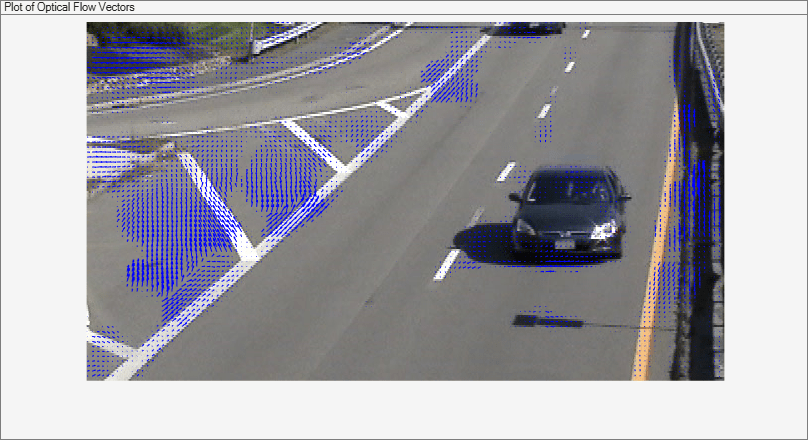# opticalFlowFarneback

Object for estimating optical flow using Farneback method

## Description

Create an optical flow object for estimating the direction and speed of moving objects using the Farneback method. Use the object function `estimateFlow` to estimate the optical flow vectors. Using the `reset` object function, you can reset the internal state of the optical flow object.

## Creation

### Syntax

``opticFlow = opticalFlowFarneback``
``opticFlow = opticalFlowFarneback(Name,Value)``

### Description

````opticFlow = opticalFlowFarneback` returns an optical flow object that you can use to estimate the direction and speed of the moving objects in a video. The optical flow is estimated using the Farneback method.```

example

````opticFlow = opticalFlowFarneback(Name,Value)` returns an optical flow object with properties specified as one or more `Name,Value` pair arguments. Any unspecified properties have default values. Enclose each property name in quotes.For example, `opticalFlowFarneback('NumPyramidLevels',3)````

## Properties

expand all

Number of pyramid layers, specified as a positive scalar. The value includes the initial image as one of the layers. When you set this value to `1`, the function estimates optical flow only from the original image frame and does not perform pyramid decomposition. The recommended values are between `1` and `4`.

Image scale, specified as a positive scalar in the range (0,1). The value specifies the rate of downsampling at each pyramid level. A value of `0.5` creates a classical pyramid, where the resolution of the pyramid reduces by a factor of two at each level. The lowest level in the pyramid has the highest resolution.

Number of search iterations per pyramid level, specified as a positive integer. The Farneback algorithm performs an iterative search for the key points at each pyramid level, until convergence.

Size of the pixel neighborhood, specified as a positive integer. Increase the neighborhood size to increase blurred motion. The blur motion yields a more robust estimation of optical flow. A typical value for `NeighborhoodSize` is `5` or `7`.

Averaging filter size, specified as a positive integer in the range [2, Inf). After the algorithm computes the displacement (flow), the averaging over neighborhoods is done using a Gaussian filter of size (`FilterSize` * `FilterSize`). Also, the pixels close to the borders are given a reduced weight because the algorithm assumes that the polynomial expansion coefficients are less reliable there. Increasing the filter size increases the robustness of the algorithm to image noise. The larger the filter size, the greater the algorithm handles image noise and fast motion detection, making it more robust.

## Object Functions

 `estimateFlow` Estimate optical flow `reset` Reset the internal state of the optical flow estimation object

## Examples

collapse all

Read a video file. Specify the timestamp of the frame to be read.

`vidReader = VideoReader('visiontraffic.avi','CurrentTime',11);`

Create an optical flow object for estimating the optical flow using Farneback method. The output is an object specifying the optical flow estimation method and its properties.

`opticFlow = opticalFlowFarneback`
```opticFlow = opticalFlowFarneback with properties: NumPyramidLevels: 3 PyramidScale: 0.5000 NumIterations: 3 NeighborhoodSize: 5 FilterSize: 15 ```

Create a custom figure window to visualize the optical flow vectors.

```h = figure; movegui(h); hViewPanel = uipanel(h,'Position',[0 0 1 1],'Title','Plot of Optical Flow Vectors'); hPlot = axes(hViewPanel);```

Read the image frames and convert to grayscale images. Estimate the optical flow from consecutive image frames. Display the current image frame and plot the optical flow vectors as quiver plot.

```while hasFrame(vidReader) frameRGB = readFrame(vidReader); frameGray = rgb2gray(frameRGB); flow = estimateFlow(opticFlow,frameGray); imshow(frameRGB) hold on plot(flow,'DecimationFactor',[5 5],'ScaleFactor',2,'Parent',hPlot); hold off pause(10^-3) end```## Algorithms

The Farneback algorithm generates an image pyramid, where each level has a lower resolution compared to the previous level. When you select a pyramid level greater than 1, the algorithm can track the points at multiple levels of resolution, starting at the lowest level. Increasing the number of pyramid levels enables the algorithm to handle larger displacements of points between frames. However, the number of computations also increases. The diagram shows an image pyramid with three levels.The tracking begins in the lowest resolution level, and continues until convergence. The point locations detected at a level are propagated as keypoints for the succeeding level. In this way, the algorithm refines the tracking with each level. The pyramid decomposition enables the algorithm to handle large pixel motions, which can be distances greater than the neighborhood size.

 Farneback, G. “Two-Frame Motion Estimation Based on Polynomial Expansion.” In Proceedings of the 13th Scandinavian Conference on Image Analysis, 363 - 370. Halmstad, Sweden: SCIA, 2003.